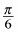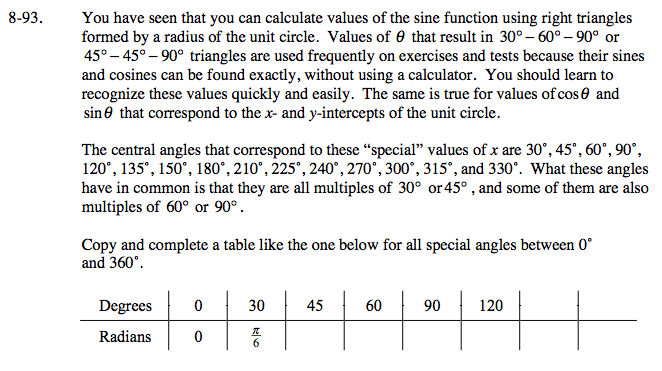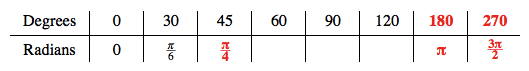Home > A2C > Chapter 8 > Lesson 8.1.6 > Problem8-93

8-93.

You have seen that you can calculate values of the sine function using right triangles formed by a radius of the unit circle. Values of θ that result in 30° − 60° − 90° or 45° − 45° − 90° triangles are used frequently on exercises and tests because their sines and cosines can be found exactly, without using a calculator. You should learn to recognize these values quickly and easily. The same is true for values of cosθ and sinθ that correspond to the x- and y-intercepts of the unit circle.

The central angles that correspond to these “special” values of x are 30°, 45°, 60°, 90°, 120°, 135°, 150°, 180°, 210°, 225°, 240°, 270°, 300°, 315°, and 330°. What these angles have in common is that they are all multiples of 30° or 45°, and some of them are also multiples of 60° or 90°.
Copy and complete a table like the one below for all special angles between 0° and 360°. Homework Help ✎

 Degrees 0 30 45 60 90 120 Radians 0$\text{Recall\:that}\:180^\circ\:\text{is}\:\pi\:\text{radians.\:\:Since}\:45\:\text{is\:one-fourth\:of}\:180,\:45^\circ\:\text{is\:one-fourth\:of}\:\pi,\:\text{or}\:\frac{\pi}{4}.$

All of the 'special' values of x are multiples of either 30° or 45°.
Knowing just these two values allows you to easily find the rest.

$\text{For example, } 225 \div 45 = 5. \text{ So } 225° = 5 \left( \frac{\pi}{4} \right) = \frac{5 \pi}{4} .$